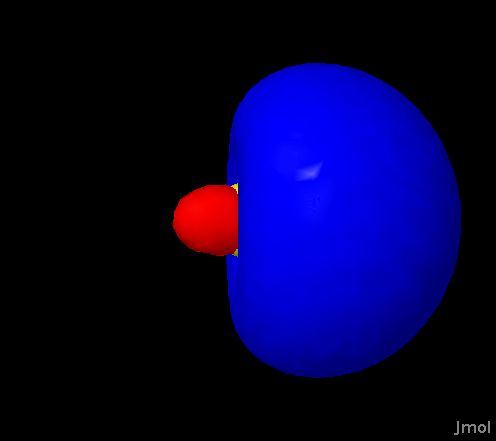Dr. Gutow's Hybrid Atomic Orbital Site
What are Hybrid Orbitals?
Hybrid orbitals are the result of a model which combines atomic orbitals on a single atom in ways that lead to a new set of orbitals that  have geometries appropariate to form bonds in the directions predicted by the VSEPR model.  The VSEPR model predicts geometries that are very close to those seen in real molecules.  There are many descriptions of hybrid orbitals available. See any general or organic chemistry text. A web search will provide additional descriptions.

First, let us see the problem with the normal s and p orbitals on an atom.  Consider methane (CH4), which consists of a carbon atom with four hydrogens arranged in a tetrahedral geometry around the cental atom.  In its ground state (lowest energy) configuration the carbon atom has an electronic configuration of [He]2s22p2.  This means that the valence electrons (the ones involved in bonding) occupy s and p orbitals.  In the figure below the 2s and the three 2p orbitals are shown superimposed on a methane molecule.  Notice that none of the orbitals point towards the hydrogens!  If we were to rotate the orbitals we would find that at most one p orbital could point towards a hydrogen at any given time.
Figure 1: A methane molecule with the carbon s and p orbitals shown.  Note that these orbitals do not point along the C-H bonds. Each of the p orbitals points along the X, Y or Z axis.

You may click on each of these four images to enlarge them.  Use your back button to return here.

To view live images of the unhybridized atomic orbitals you can go to Dr. Gutow's atomic orbital page.  To compare with hybrid orbitals use these links for sp, sp2 and sp3 hybrids.
This means these orbitals as we usually visualize them cannot take part in the bonding.  The solution is to combine these orbitals (add and subtract them from each other) to get four orbitals pointing in the proper direction.  To get four new orbitals we have to combine four atomic orbitals.  Thus the orbitals that point the correct directions are made by combining the 2s and all three of the 2p orbitals.  This kind of hybrid is called an sp3 hybrid orbital and is the kind of hybridization seen in molecules of tetrahedral geometry.

How the atomic orbitals are combined to give sp3 orbitals is a bit complicated, so we will consider the simpler case of the sp hybrid formed from combining a single s and p orbital.  This hybridization is what is seen for carbon in linear molecules like CO2 and acetylene (C2H2).  To form separate bonds pointing 180 degrees away from each other two separate orbitals pointing in opposite directions are needed.  These are formed by combining the spherically symmetric s orbital with one of the p orbitals.  The p orbital by itself doesn't work because both lobes are part of the same orbital, so can only accommodate one pair of electrons.  In two bonds there are two pairs of electrons to accommodate.

In this example we will combine the 2s orbital with the 2px orbital.  Since we start with two atomic orbitals we will get two atomic orbitals.  The two combinations are 2s + 2px and 2s - 2px.  Consider the + combination.  Since the two lobes of the p orbital have opposite signs the positive lobe (+) adds to the s orbital and increases the electron density on that side of the atom.  The negative lobe (-) subtracts from the s orbital and decreases the electron density on the other side of the atom.  Since the orbitals represent electron waves the sign actually represents the phase of the waves and addition is constructive interference while subtraction is destructive interference.  The - combination causes enhancement of the electron density on the opposite side from the + combination.  This is illustrated in the graphic below.+ case:- case:sp+:sp-:Figure 2:  The left image is of both the 2s and the 2px orbital on a single atom.  The sphere in the middle represents the location of the nucleus, much enlarged so that you can see it.  The yellow mesh represents the 2s orbital with positive phase, the solid blue surface one lobe (positive) of the 2p and the red surface the other lobe (negative) of the 2p.  The middle animations show how the + (top) and - (bottom) combinations yield two orbitals pointing opposite directions.  The images at right show the two sp orbitals (sp+ and sp-).  To manipulate these orbitals and compare them directly with the other 2py and 2pz orbitals that did not get used in making the hybrids see the interactive sp hybrid page.

The other hybrids use more complicated sums (not just 50:50 combinations) of the orbitals that are combined to yield the appropriate hybrid orbitals.  You can determine the hybridization of an atom from the VSEPR geometry about that atom.  Notice that the sum of the exponents is the same as the number of groups about the atom.  This is also the same as the total number of hybrid orbitals you get.  The relationship is shown in the table below.

Table 1: Relationship of the VSEPR geometries to atomic hybridization.
 Number of Groups around the atom Sub-Shapes A = central atom X = atom attached to central atom E = nonbonding electron group on central atom Hybridization 2 AX2,  Linear sp 3 AX3, Trigonal Planar AX2E, Bent sp2 4 AX4, Tetrahedral AX3E, Trigonal Pyramidal AX2E2, Bent sp3 5 AX5, Trigonal Bipyramidal AX4E, See-Saw AX3E2, T-Shaped AX2E3Linear sp3d 6 AX6, Octahedral AX5E, Square Pyramidal AX4E2,  Square Planar sp3d2